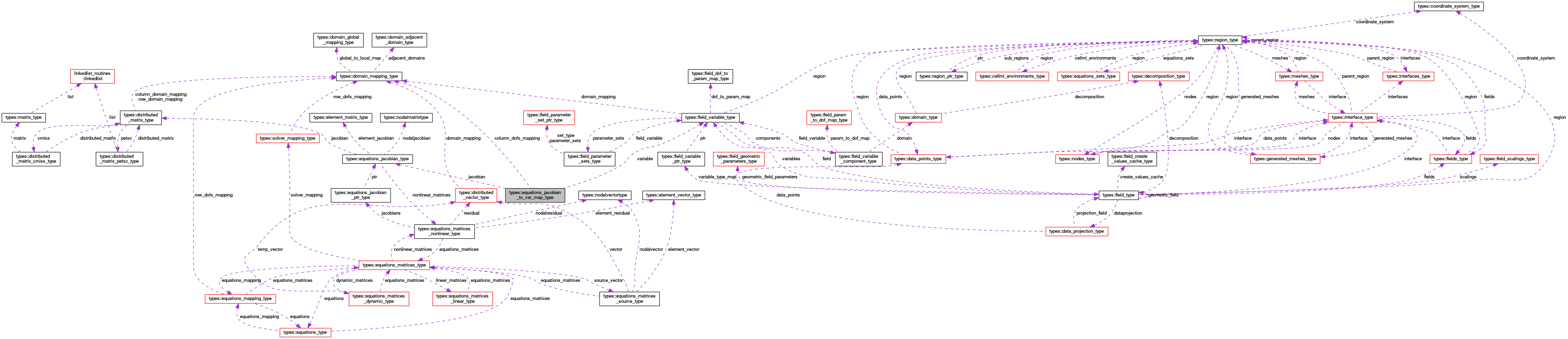OpenCMISS-Iron Internal API Documentation
types::equations_jacobian_to_var_map_type Type Reference

Contains the mapping from the Jacobian back to the nonlinear residual variables. More...

Collaboration diagram for types::equations_jacobian_to_var_map_type:[legend]

## Public Attributes

integer(intg) jacobian_number
The equations Jacobian matrix number. More...

integer(intg) variable_type
The dependent variable type mapped to this equations matrix. More...

type(field_variable_type), pointer variable
A pointer to the field variable that is mapped to this equations matrix. More...

type(equations_jacobian_type), pointer jacobian
A pointer to the equations matrix for this variable. More...

integer(intg) number_of_columns
The number of columns in this equations matrix. More...

real(dp) jacobian_coefficient
The multiplicative coefficent for the matrix in the equation set. More...

integer(intg), dimension(:), allocatable equations_column_to_dof_variable_map
COLUMN_TO_DOF_MAP(column_idx). The variable DOF that the column_idx'th column of this equations matrix is mapped to. More...

type(domain_mapping_type), pointer column_dofs_mapping
A pointer to the column dofs domain mapping for the matrix variable. More...

## Detailed Description

Contains the mapping from the Jacobian back to the nonlinear residual variables.

Definition at line 1599 of file types.f90.

## Member Data Documentation

 type(domain_mapping_type), pointer types::equations_jacobian_to_var_map_type::column_dofs_mapping

A pointer to the column dofs domain mapping for the matrix variable.

Definition at line 1607 of file types.f90.

 integer(intg), dimension(:), allocatable types::equations_jacobian_to_var_map_type::equations_column_to_dof_variable_map

COLUMN_TO_DOF_MAP(column_idx). The variable DOF that the column_idx'th column of this equations matrix is mapped to.

Definition at line 1606 of file types.f90.

 type(equations_jacobian_type), pointer types::equations_jacobian_to_var_map_type::jacobian

A pointer to the equations matrix for this variable.

Definition at line 1603 of file types.f90.

 real(dp) types::equations_jacobian_to_var_map_type::jacobian_coefficient

The multiplicative coefficent for the matrix in the equation set.

Definition at line 1605 of file types.f90.

 integer(intg) types::equations_jacobian_to_var_map_type::jacobian_number

The equations Jacobian matrix number.

Definition at line 1600 of file types.f90.

 integer(intg) types::equations_jacobian_to_var_map_type::number_of_columns

The number of columns in this equations matrix.

Definition at line 1604 of file types.f90.

 type(field_variable_type), pointer types::equations_jacobian_to_var_map_type::variable

A pointer to the field variable that is mapped to this equations matrix.

Definition at line 1602 of file types.f90.

 integer(intg) types::equations_jacobian_to_var_map_type::variable_type

The dependent variable type mapped to this equations matrix.

Definition at line 1601 of file types.f90.